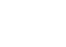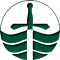### MATH 135H Assignment #2

Due: 11 October, 2000

Applying linear algebra to non-linear algebra

Consider the points (2,5), (0,1), and (3,4) in two-dimensional space.

1. Using linear algebra, find the equation of a parabola passing through all three of these points. 

2. Are there any other parabolas passing through all three of these points? If so, give an example of such a parabola and explain how many there are; if not, explain why there aren't any more. 

3. Would you be able to carry out the methods you used in 1 and 2 for any three distinct points in two dimensions? If so, explain why; if not, what additional requirement(s) would the points have to satisfy to let you do so? 

Solutions to Assignment #2Department of MathematicsTrent University